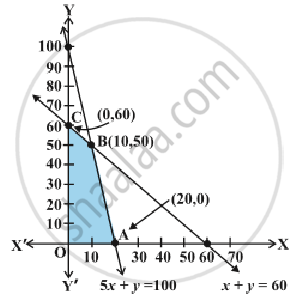HSC Science (Electronics) 12th Board ExamMaharashtra State Board
Share

# Graphical Method of Solving Linear Programming Problems

#### notes

Let us graph the constraints stated as linear inequalities:
5x + y ≤ 100 ... (1)
x + y ≤ 60 ... (2)
x ≥ 0 ... (3)
y ≥ 0 ... (4)
The graph of this system (shaded region) consists of the points common to all half planes determined by the inequalities (1) to (4) in following fig.Each point in this region represents a feasible choice open to the dealer for investing in tables and chairs. The region, therefore, is called the feasible region for the problem. Every point of this region is called a feasible solution to the problem. The region other than feasible region is called an infeasible region.
The point   (10, 50) (0, 60), (20, 0) is a feasible solution of the problem. Any point outside the feasible region is called an  infeasible solution. For example, the point (25, 40) is an infeasible solution of the problem.

Optimal (feasible) solution:
Any point in the feasible region that gives the optimal value (maximum or minimum) of the objective function is called an optimal solution. Every point in the feasible region satisfies all the constraints and since there are  infinitely many points, we can find the optimal solution using fundamental theorms in Linear programming problems.

#### theorem

Let R be the feasible region (convex polygon) for a linear programming problem and let Z = ax + by be the objective function. When Z has an optimal value (maximum or minimum), where the variables x and y are subject to constraints described by linear inequalities, this optimal value must occur at a corner point* (vertex) of the feasible region.

#### theorem

Let R be the feasible region for a linear programming problem, and let Z = ax + by be the objective function. If R is bounded, then the objective function Z has both a maximum and a minimum value on R and each of these occurs at a corner point (vertex) of R.

If R is unbounded , then a maximum or a minimum value of the objective function may not exist.
Corner points of the bounded (feasible) region :
O(0,0)  A(20,0) B(10,50) C(0,60)
Computing the values of Z = 250x + 75 y at these points -

 Vertex of the feasible region Corresponding value of   Z  = 250 x +75 y (in Rs.) O(0,0) 250(0) + 75(0) = 0 A(20,0) 250(20)+75(0) = 5000 B(10,50) 250(10)+75(50) = 6250   ←  maximum C(0,60) 250(0)+75(60)=4500

We observe that the maximum profit to the dealer results from the investment strategy (10, 50), i.e. buying 10 tables and 50 chairs.

This method of solving linear programming problem is referred as Corner Point Method.
The method comprises of the following steps :

1. Find the feasible region of the linear programming problem and determine its corner points (vertices) either by inspection or by solving the two equations of the lines intersecting at that point.

2. Evaluate the objective function Z = ax + by at each corner point. Let M and m, respectively denote the largest and smallest values of these points.

3. (i) When the feasible region is bounded, M and m are the maximum and minimum values of Z.
(ii) In case, the feasible region is unbounded, we have:

4. (a) M is the maximum value of Z, if the open half plane determined by ax + by > M has no point in common with the feasible region. Otherwise, Z has no maximum value.

(b) Similarly, m is the minimum value of Z,if the open half plane determined by ax + by < m has no point in common with the feasible region. Otherwise, Z has no minimum value.

### Shaalaa.com

Linear Programming part 2 (Graphical Method Thoerem 1) [00:12:56]
S
series 1
0%

S